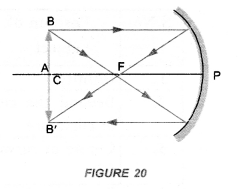Chapter 10 Light Reflection and Refraction Class 10 Science Important Questions with Answers PDF will help you in scoring more marks. This consists of 1 mark Questions, 3 Mark Numericals Questions, 5 Marks Numerical Questions and previous year questions from Chemical Reactions and Equations Chapter.

## Light Reflection and Refraction Class 10 Important Questions and Answers Science Chapter 10

Very Short Answer Questions (1 Mark)

Based on Reflection of light

Question 1.
Define reflection of light ?
The process of returning or bouncing back the light to the same medium after striking the surface is called reflection of light.

More Resources

Question 2.
What is a reflector ?
A surface which reflects the light is called reflector.

Question 3.
State laws of reflection.

1. Angle of incidence is equal to the angle of reflection. That is , ∠i = ∠r .
2. Incident ray, reflected ray and normal to the reflecting surface at the point of incidence lie in the same plane.

Question 4.
What are the values of angle of incidence ∠i and angle of reflection ∠r for normal incidence of light on a plane mirror ? [CBSE (All India) 2005]
For normal incidence, ∠i = 0. Therefore, according to law of reflection ∠r = ∠i = 0.

Question 5.
What is real image ?
When rays of light from an object actually meet at a point after refraction, then image formed is real.

Question 6.
What is virtual image ?
When rays of light from an object do not meet at a point but appears to meet at a point, then image formed is virtual.

Question 7.
Mention the nature of image produced by a plane mirror. (CBSE 2014)
Image is virtual, erect and of the same size as that of the object.

Based on curved or spherical mirrors

Question 8.
Define centre of curvature of a spherical mirror.
The centre of a hollow sphere of which the spherical mirror forms a part is called the centre of curvature of the spherical mirror.

Question 9.
Define radius of curvature of a spherical mirror.
The radius of a hollow sphere of which the spherical mirror forms a part is called radius of curvature of the spherical mirror.

Question 10.
Define aperture of a spherical mirror.
The part of spherical mirror exposed to the incident light is called the aperture of the spherical mirror.

Question 11.
Define pole of a spherical mirror.
The mid point of the spherical mirror is called the pole of spherical mirror.

Question 12.
Define principal axis of the spherical mirror.
The line joining the centre of curvature and pole of the spherical mirror and extended on either side is called the principal axis of the spherical mirror.

Question 13.
Define the principal focus of a convex mirror.
A point on the principal axis, where rays of light parallel to the principal axis appear to meet after reflecting from the convex mirror.

Question 14.
Define the principal focus of a concave mirror.
A point on the principal axis, where the rays of light parallel to the principal axis meet after reflection from the concave mirror is called the principal focus of the concave mirror.

Question 15.
Define focal length of a spherical mirror.
The distance between the pole and principal focus of a spherical mirror is called focal length of the spherical mirror.

Question 16.
Write down the relation between the focal length (f) and radius of curvature (R) of a spherical mirror.
f = R/2.

Question 17.
Name the mirror which acts as
(i) a convergent mirror and
(ii) divergent mirror.
(i) concave mirror,
(ii) convex mirror.

Question 18.
Copy the figure in your answer-book and show the direction of light after reflection. [CBSE (Delhi) 2008]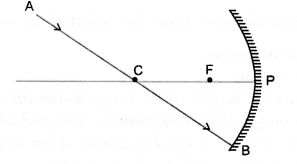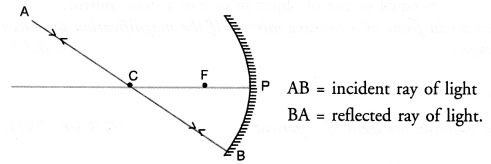Question 19.
Copy the figure in your answer-book and show the direction of light after reflection. Also write the angle of reflection.Question 20.
No matter how far you stand from a mirror, your image always appears erect and diminished. Identify the type of mirror. [CBSE (All India) 2005; CBSE (Foreign) 2016]
Or
If the image formed by a mirror for all positions of the object placed in front of it is always diminished, erect and virtual. State the type of mirror. (CBSE 2017)
Convex mirror.

Question 21.
Where should an object be placed on the principal axis of a concave mirror so that its real image is of the same size as that of the object ?
Object should be placed at the centre of curvature of the concave mirror.

Question 22.
Where should an object be placed on the principal axis of a concave mirror so that a magnified and virtual image of the object is formed ?
Object must be placed between the pole and focus of the concave mirror.

Question 23.
Where will the image be formed by a convex mirror if object is placed between infinity and pole of the mirror r.
Between pole and focus of the convex mirror and behind the convex mirror.

Question 24.
Name a mirror that gives an erect and magnified (enlarged) image of an object.
Concave mirror.

Question 25.
Write mirror formula.
Or
Write relation between u,v and ffor mirrors. (CBSE 2014, 2016)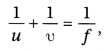where u is the object distance from the spherical mirror, v is the image distance from the mirror and f is the focal length of the mirror.

Question 26.
Define linear magnification of a mirror.
It h defined as the ratio of the size (or height) of the image to the size (or height) of the object.

Question 27.
What is the magnification of the images formed by plane mirrors and why ? (CBSE 2015)
Magnification, m = 1 because size of image is equal to size of object in case of a plane mirror.

Question 28.
An object of size 2 cm is placed at 25 cm in front of a concave mirror. If the magnification produced by the mirror is 4, what is the size of the image ?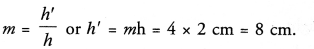Question 29.
Name the mirror used by a dentist to examine the teeth of a patient.
Concave mirror.

Question 30.
Name the rear view mirror used in vehicles.
Or
Which type of mirror is usually used as a rear-view mirror in motor-cars ?
[CBSE (Foreign) 2008, CBSE 2011]
Convex mirror.

Question 31.
Name the type of mirrors used in head lights of buses and cars. (CBSE 2012)
Or
Which kind of mirrors are used in the head lights of a motor-car and why ?
Concave mirrors. This is because these mirrors send the reflected light as a parallel beam of light to illuminate the road.

Question 32.
Name the type of mirror used in solar furnaces. (CBSE 2013)
Concave mirror.

Question 33.
Why does a ray of light passing through the centre of curvature of a concave mirror after reflection, is reflected back along the same path ? (CBSE 2011)
A ray of light passing through the centre of curvature of a concave mirror falls perpendicular to the surface of the mirror i.e. ∠i = 0. According to the law of reflection ∠r = ∠i = 0. Therefore, the ray is reflected along the same path.

Question 34.
What kind of mirrors are used in big shopping stores to watch the activities of customers ? [CBSE (All India) 2009)]
Convex mirrors.

Question 35.
State the relation between the height of an object (h), height of image (h) object, distance (u) and image distance (v) in case of a spherical mirror.Question 36.
In the following diagram, MM’ is a concave mirror and AB is an object. Draw on your answer-sheet a ray diagram to show the formation of image of this object.
[CBSE (Foreign) 2017]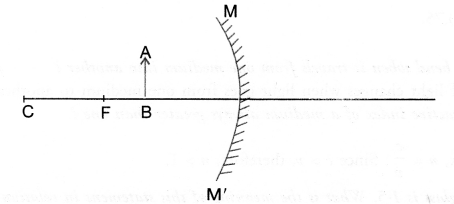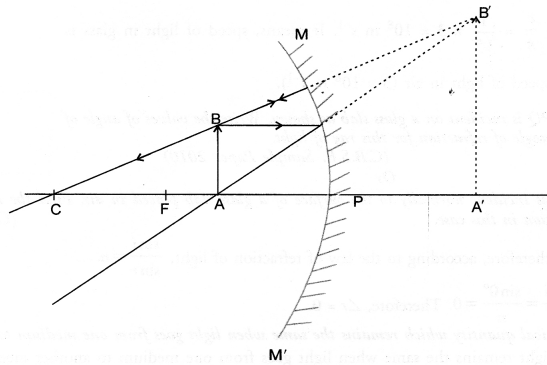Based on refraction of light

Question 37.
What is refraction of light ?
The bending of light when it passes obliquely from one medium to light.

Question 38.
What is the cause of refraction of light ?
The refraction of light is due to the fact that speed of light is different in different media.

Question 39.
Define refractive index and express it mathematically. (CBSE 2011, 2012)
Or
Define absolute refractive index of a medium. (CBSE 2012, 2013, 2015, 2016)
Refractive index is defined as the ratio of the speed of light in vacuum (c) to the speed of light in medium (v).
That is, n = c/v .

Question 40.
Define relative refractive index of a medium.
Relative refractive index of a medium (2) w.r.t. medium (1) is defined as the ratio of speed of light in medium (1) to the speed of light in medium (2).

Question 41.
Write Snell’s law. (CBSE 2011)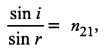where i = incident angle, r = refracted angle and n21 = constant and is the refractive index of medium 2 with respect to medium 1.

Question 42.
If refractive index of water for light going from air to water is 1.33, what will be the refractive index of air for light going from water to air ? (CBSE 2011)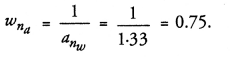Question 43.
Why does a ray of light bend when it travels from one medium into another ? [CBSE (Delhi) 2009]
This is because speed of light changes when light goes from one medium to another medium.

Question 44.
Why is the absolute refractive index of a medium always greater than one ? (CBSE 2011, 2016)
Absolute refractive index, n = c/v . Since c > v, therefore, n > 1.

Question 45.
The refractive index of glass is 1.5. What is the meaning of this statement in relation to speed of light ?
[CBSE (Delhi) 2008]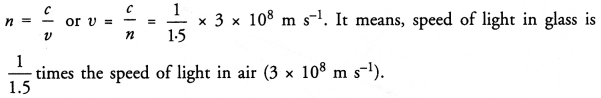Question 46.
A ray of light PQ is incident on a glass slab as shown. Write the values of angle of incidence and angle of refraction for this ray of light.
(CBSE Sample Paper 2010)Or
A ray of light is incident normally to the surface of a glass slab placed in air. Find the angle of incident and angle of refraction in this case. (CBSE 2011, 2013)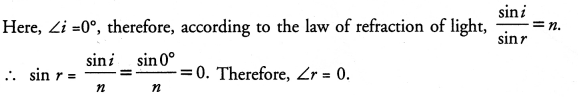Question 47.
Name the physical quantity which remains the same when light goes from one medium to another medium.
Frequency of light remains the same when light goes from one medium to another medium.

Question 48.
What happens when a ray of light strikes the surface of separation between the two media at right angle ? (CBSE 2011)
There is no refraction of light and the ray of light continues to travel along the same path.

Question 49.
What is the angle between the incident ray of light and the emergent ray of light, when incident ray of light falls obliquely on the glass slab ?
When incident ray of light falls obliquely on the glass slab, then the emergent ray of light is parallel to the incident ray of light. Therefore, angle between the incident and emergent rays of light is zero.
A ray of light falls obliquely on the glass slab. The perpendicular distance between the emergent ray of light and the original path of the incident ray is 2 mm.

Question 50.
What is the lateral displacement of the ray of light ?
Lateral displacement of the ray of light = 2 mm. on Refraction by spherical lens

Question 51.
What do you understand by optical centre of a lens ?
A point in a lens through which rays of light pass without deviation in their original path.

Question 52.
Define principal axis of a lens.
A line joining the centres of curvature of two spherical surfaces forming a lens is called principal axis of the lens.

Question 53.
Define principal focus of a convex lens.
A point on the principal axis of a convex lens where all rays of light parallel to the principal axis meet after refracting through the lens is called principal focus of the convex lens.

Question 54.
Define principal focus of a concave lens.
A point on the principal axis of a concave lens where all rays of light parallel to the principal axis appear to meet after refracting through the lens is called principal axis of the concave lens.

Question 55.
Define focal length of a lens. [CBSE (All India) 2005]
The distance between the optical centre and principal focus of a lens is called the focal length of the lens.

Question 56.
Write lens formula.
Or
Write relation between u, v and ffor a lens. (CBSE 2014, 2016)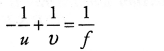Question 57.
State the relation between the height of an object (h), height of image (h), object distance (u) and image distance (v) in case of a lens. (CBSE 2011)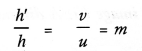Question 58.
Incident ray AB falls on a lens. The refracted ray is BC as shown in figure. Name the lens.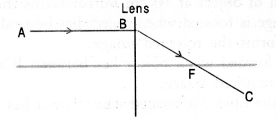Since the ray AB converges along BC after passing

Question 59.
Incident ray AB falls on a lens. The refracted ray BC is shown in figure. Name the lens.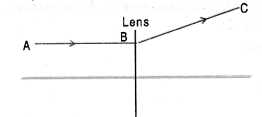Since the ray AB diverges along BC after passing through the lens, so the lens is diverging or concave lens.

Question 60.
If on applying cartesian sign conventions for spherical lenses, the image distance obtained is negative, state the significance of the negative sign. (CBSE 2011)
Negative sign with image distance signifies that image is formed on the same side in which the object lies or image is formed in front of the lens.

Question 61.
Define power of a lens and write its SI unit. [CBSE Saniple Paper 2010, 2012, 2015, 2016)]
Power of a lens is its ability to converge or diverge the rays of light falling on it. Power of a lens is equal to reciprocal of the focal length of the lens.
SI unit of power is dioptre (D).

Question 62.
In what SI unit is the power of lenses stated ? [CBSE (Foreign ) 2005)]
Dioptre (D).

Question 63.
A convex lens forms a virtual and magnified image. What should he the position of the object on the principal axis ?
Between focus and the optical centre of the lens.

Question 64.
State the relation between the focal length (f) and power of a lens.
Or
How are power and focal length of a lens related ? [NCERT Question Bank; CBSE 2016]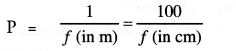Question 65.
A lens has -4D power. Is the lens concave or convex ?
Lens is concave.

Short Answer Questions( 2 & 3 Marks)

Based on Reflection of Light

Question 1.
List two differences between real and virtual images

 S.No. Real Image Virtual Image 1. When rays of light after reflection meet at a When rays of light after reflection do not meet at a point point, real image is formed. but appears to meet at a point, virtual image is formed. 2. Real image can be obtained on a screen. Virtual image cannot be obtained on a screen. 3. Real image is formed in front of a mirror. Virtual image is formed behind the mirror. 4. Real image is always inverted. Virtual image is always erect {i.e., upright).

Question 2.
State the laws of reflection of light.

1. Angle of incidence is equal to the angle of reflection. That is , ∠i = ∠r .
2. Incident ray, reflected ray and normal to the reflecting surface at the point of incidence lie in the same plane.

Based on curved or spherical mirror

Question 3.
With the ray diagram show that angle of incidence is equal to the angle of reflection when a ray is incident on the concave mirror. (CBSE 2015)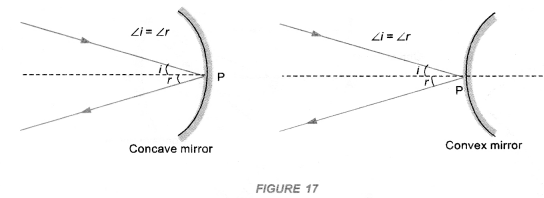Question 4.
An object is placed at the following distances from a concave mirror of focal length 15 cm.
(a) 10 cm
(b) 20 cm
(c) 30 cm
(d) 40 cm
Which position of the object will produce

1. Virtual image
2. A diminished real image
3. An enlarged real image
4. An image of same size. (CBSE 2011, 2012)

1. Concave mirror forms virtual image if object is placed between the focus and pole of the mirror. Therefore, for the position of object at 10 cm mirror forms the required image.
2. A real and diminished image is formed when object lies beyond C i.e. beyond 2F. So, for the position of object at 40 cm, mirror forms the required image.
3. An enlarged real image is formed when object lies between F and 2 F. So, for the position of object at 20 cm, mirror forms the required image.
4. An image of same size of the object is formed when object lies at C or 2F. So, for the position of object at 30 cm, mirror forms the required image.

Question 5.
Draw ray diagram to show the formation of images when the object is placed in front of a concave mirror
(i) between its pole and focus point,
(ii) on the centre of curvature. (CBSE 2014, 2016)
(i)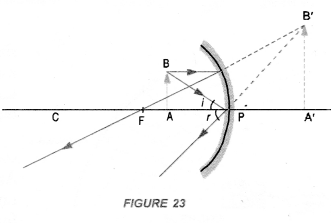(ii)Question 6.
State three uses of a concave mirror. (CBSE 2012)

1. Reflector: Concave mirrors are used in motor head lights, search lights and torches etc. to produce an intense parallel beam of light. A bulb is placed at the focus of concave mirror or concave reflecting surface. The beam of light from the bulb after reflecting from the concave mirror goes as a parallel beam (figure 24).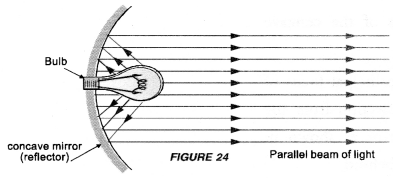This parallel beam of – light illuminates the road ahead of the vehicle.
2. Shaving and make up mirror: When an object is placed close to a concave mirror (i.e between the pole and focus of the concave mirror), an erect and enlarged (large in
size) image of the object is formed. Because of this fact, concave mirror is used by men to see their enlarged faces while shaving. Similarly, a lady can see her face better with the help of a concave mirror while doing make up.
3. In solar cookers: When a parallel beam of sun light falls on a concave mirror, this beam is brought to the focus of the concave mirror. As a result of this, the temperature of an object (say a container containing un-cooked food) placed at this focus increases considerably. Hence the food in the container is cooked (figure 25).Question 7.
State two uses of a convex mirror. (CBSE 2012)

1. Rear view or driver’s mirror. Convex mirror is used as a rear view mirror in vehicles because this mirror forms an erect and diminished image of an object behind the vehicle. Since the image of the object formed is small in size, so the field of view is increased. It means, the driver of a vehicle can see the traffic over large area behind his vehicle. This mirror is also known as driver’s mirror.
2. In street lights. Convex mirror is used in street lights to diverge light over a large area (figure 28).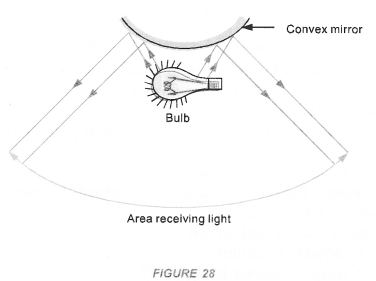Question 8.
Parallel rays of light incident on a concave mirror and a convex mirror as shown in figure,
(i) Redraw the reflected rays in both the cases,
(ii) Name the points where the reflected rays meet or appear to meet on the principal axis.(i) Reflected rays are shown in figures.(ii) The point where the reflected rays of light meet or appear to meet on the principal axis is known as principal focus F of the concave mirror or convex mirror.

Question 9.
A ray of light LM is incident on a mirror as shown in the figure. The angle of incidence for this ray is the angle between it and the line joining two other points in the figure. Name these two points.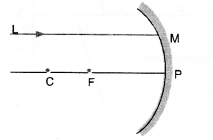The angle of incidence for a ray falling on a spherical mirror is the angle between the incident ray and the normal to the point of incidence. A line joining the centre of curvature and the point of incidence is a normal to the point of incidence. Thus, the given two points are C and M as shown in figure.Question 10.
A ray of light AM is incident on a spherical mirror as shown in the diagram. Redraw the diagram and show the reflected ray. Also indicate and mark the angle of incidence and angle of reflection in the diagram. (CBSE 2012)Or
Draw a ray diagram to show the path of the reflected ray corresponding to an incident ray of light parallel to the principal axis of a concave mirror. Mark the angle of incidence and angle of reflection on it.
(CBSE 2014, 2017)
Incident ray AM is parallel to the principal axis of a concave mirror. Hence, it will pass through the principal focus (F) of the mirror after reflection as shown in figure.Question 11.
Incident ray AB passes through the focus (F) of a concave mirror and strikes the mirror at B as shown is figure. Trace the reflected ray and also mark the angle of incidence and angle of reflection in the figure. (CBSE 2012)
Or
Copy the figure in your answer-book and show the direction of the light after reflection.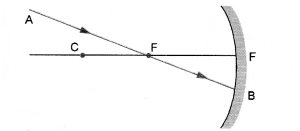Incident ray passing through the focus (F) of a concave mirror goes parallel to the principal axis after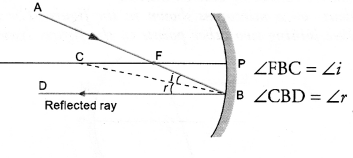Question 12.
Draw the ray diagram to show reflected ray by a concave mirror when the incident ray
(a) passes through its centre of curvature
(b) passes through its focus
(c) is incident on pole of the concave mirror. (CBSE 2015)
(a)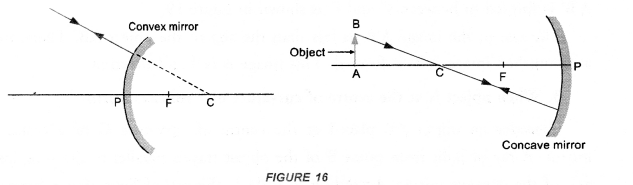(b)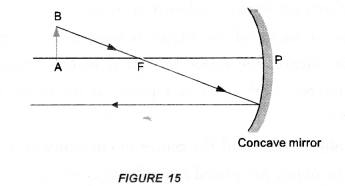(c)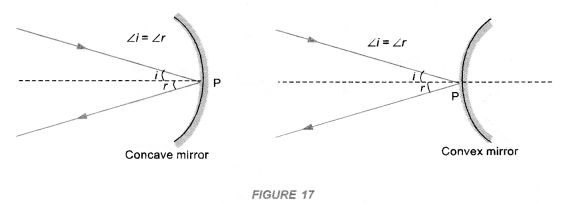Question 13.
Name the type of mirror used in a solar furnace. How is high temperature achieved by this device ?
(CBSE 2011, 2013)
Concave mirror. The sun rays are focused at a point by the concave mirror. The concentrated beam of sun light at a point increases the temperature at that point.

Question 14.
Draw ray diagrams to describe the nature, position and relative size of the image formed by a concave mirror for the object
(i) When the object is placed between the centre of curvature and the focus of the concave mirror,
(ii) When the object is placed between the pole and the focus of the concave mirror.
(CBSE 2004, 2011, 2012, 2013)
(i)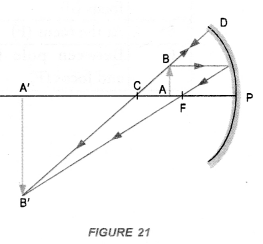(ii)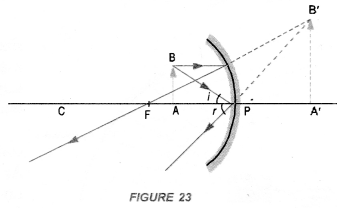Question 15.
A spherical mirror produces an image of magnification -1.0 on a screen placed at a distance of 30 cm from the pole of the mirror.
(i) Write the type of mirror in this case.
(ii) What is the focal length of the mirror ?
(iii) What is the nature of the image formed ?
(iv) Draw the ray diagram to show the image formation in this case. (CBSE 2014)
(i) Concave mirror(iii) Image is real, inverted and of the same size as that of the object.
(iv)Question 16.
The magnification produced by a mirror is -1.5. What does it signify about the image formed ?
(CBSE 2011)It means, size of image =1.5 times the size of the object. Negative sign signifies that the image is inverted. Inverted image is real image.

Question 17.
Identify the nature of the mirror and mention two characteristics of the image formed when magnification (m) = + 6. (CBSE 2011)
m = + 6 means size of image formed = 6 times the size of the object and the image is erect. Such image is formed by a concave mirror when object is placed between the focus and pole of the mirror. The image is virtual and magnified.

Question 18.
Mention two properties of image formed by a convex mirror. (CBSE 2011)
Image formed by a convex mirror is

1. virtual and erect,
2. smaller in size as compared to the size of the object.

Question 19.
Why does a ray of light passing through the centre of curvature of a concave mirror after reflection, is reflected back along the same path ? (CBSE 2011)
A ray of light passing through the centre of curvature of the concave mirror strikes the surface of the mirror . normally i.e. ∠i = 0. According to the law of reflection, ∠r = ∠i = 0.
Thus, the reflected ray retraces its path.

Question 20.
How can you identify the three types of mirrors without touching ? (CBSE 2011)
We can identify the three types of mirrors by looking at them. If the size of the image of an object is of the
same size as that of the object, then mirror is plane mirror. If the size of the image of an object increases as the object is brought closer to the mirror and the size of image is highly magnified when the object is very close to the mirror, then the mirror is concave mirror. If the size of the image is smaller and full length of the image of an object is seen, then the mirror is convex mirror.

Question 21.
Name the mirror that
(a) can give real as well as virtual image of an object
(b) will always give virtual image of same size of an object
(c) will alway give virtual and diminished image of an object
(d) is used by a doctor in examining teeth. (CBSE 2011)
(a) Concave mirror
(b) Plane mirror
(c) Convex mirror
(d) Concave mirror.

Question 22.
What should be the position of the object, when a concave mirror is to be used :
(i) as a shaving mirror, and
(ii) in torches producing parallel beam of light ? (CBSE 2011, 2012)
(i) between the focus and pole of the concave mirror
(ii) at the focus of the concave mirror.

Question 23.
A man standing in front of a mirror finds his image having a very large head and legs of normal size. What type of mirrors are used in designing such a mirror ? (CBSE 2011)
The upper part of such a mirror is concave mirror and lower part is a plane mirror.

Question 24.
A concave mirror of focal length ft can form a magnified erect as well as an inverted image of an object
placed in front of it. ” Justify this statement stating the position of the object with respect to the mirror in each case for obtaining these images. (CBSE 2013)

1. When object is placed between the focus (F) and pole (P) of a concave mirror, magnified and erect image of the object is formed on the back of the mirror.
2. When object is placed between the centre of curvature and the principal focus of a concave mirror, magnified and inverted image is formed in front of the mirror.

Based on Refraction of light

Question 25.
State laws of refraction of light. [CBSE (Foreign) 2004, 2012, 2013, 2014, 2015]
Refraction of light follows the following two laws :

1. The incident ray, the refracted ray and the normal to the surface separating the two media all lie in the same plane.2. The ratio of the sine of the incident angle (∠i) to the sine of the refracted angle (∠r) is constant for a pair of two media.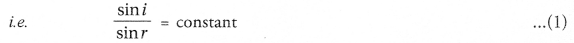This constant is known as the refractive index of the medium in which refracted ray travels with respect to the medium in which incident ray travels. Refractive index is denoted by n.
3. Refractive index of second medium with respect to the first medium is denoted by
Thus, eqn. (1) can be written as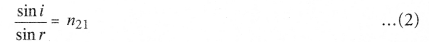This law is also known as Snell’s law as it was stated by Prof. Willebrord Snell (Dutch mathematician and astronomer).

Question 26.
Prove that if a ray of light enters a rectangular glass slab obliquely and emerges from the opposite face of the glass slab, the emergent ray will be parallel to the incident ray. [CBSE (Foreign) 2004]
Or
(a) With the help of a diagram, show that when light falls obliquely on a side of a rectangular glass slab, the emergent ray is parallel to the incident ray. (CBSE 2013)
(b) Show the lateral displacement of the ray on the diagram. (CBSE 2008)
Perform an experiment to demonstrate that light bends from its path, when it falls obliquely on the surface of a glass slab. Also show that angle of incidence is about equal to the emergent angle.

1. Take a glass slab and place it on a white sheet of paper fixed on a drawing board.
2. Mark the boundary ABCD of the glass slab.
3. Fix two pins P1 and P2, vertically on the drawing board such that line joining the pins is inclined to the edge AB of the glass slab (Figure 37).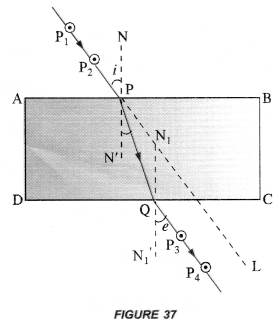4. Now, look through the glass slab from opposite side so that the images of pins P1 and P2 are seen exactly in line. Fix two pins P3 and P4, vertically on the drawing board such that pins P3, P4 and image of P1 and P2 are exactly in one line.
5. Remove the glass slab and all pins. Join the points P1 and P2 with a line and extend this line to touch the edge AB at P. Similarly join the points P3 and P4 with a line and extend the line to touch the edge DC at Q.
6. Join points P and Q with a straight line.
7. Draw normal NN’ at P on the edge AB and a normal N1N1‘ at Q on the edge DC.
8. Measure ∠P2 PN and ∠P3 QN1‘ using a protractor or dee.

This experiment shows that when light falls obliquely on a glass slab, it bends along PQ from its original path along PL.
Also ∠P2PN ≈ ∠P3QN1‘. That is, angle of incidence is about equal to the emergent angle.
Note : Distance between pins P1 and P2, and between P3 and P4 must be large.

Question 27.
For the same angle of incidence 45°, the angle of refraction in two transparent media : I and II is 20° and 30° respectively. Out of I and II, which medium is optically denser and why ? (CBSE 2011)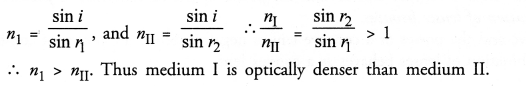Question 28.
A ray of light falls normally on the surface of a transparent glass slab. Draw a ray diagram to show its path and also mark angle of incidence and angle of emergence. (CBSE 2013)
Angle of incidence = 0, angle of emergence = 0.

Based on Refraction by spherical lens

Question 29.
What is the difference between a convex lens (converging lens) and a concave lens (diverging lens) ?
(CBSE 2012)

 Convex lens Concave lens 1.  It is thick in the middle and thin at edges. 1.  It is thin in middle and thick at edges. 2.  When placed close to eye, it forms magnified image of an object. For example, if you place it close to your book, the words will appear large in size. 2.    It forms diminished image of an object. If you place it close to your book, the words will appear small in size.

Question 30.
Study the ray diagram given below and answer the following questions :(i)State the type of lens used in the figure.
(ii) List two properties of the image formed.
(iii) In which position of the object will the magnification be – 1 ? (Sample Paper 2012-13)
(i) Lens is convex lens.
(ii) Image formed is real, inverted and enlarged.If object is placed at 2F1 Size of image = Size of object, then in this case, m = – 1.

Question 31.
Draw ray-diagrams to describe the nature, position and relative size of the image formed
(i) by a convex lens for the object when it is placed at 2F1
(ii) by a concave mirror for the object when it is placed between pole P and focus F.
(CBSE 2008, 2009, 2011, 2016)
(i)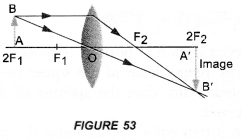(ii)Question 32.
Draw ray diagrams to describe the nature, position and’relative size of the image formed by a convex lens for the object
(i) When the object is placed at F1 and
(ii) When the object is placed between F1 and optical centre of the lens. (CBSE 2011, 2016)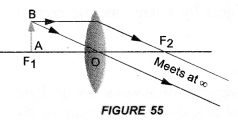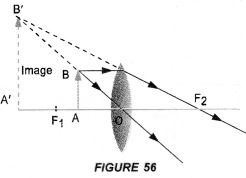Question 33.
Draw ray diagrams to describe the nature, position and relative size of the image formed by a concave lens for the object
(i) When the object is placed at infinity and
(ii) When the object is placed to between infinity and optical centre of the lens. (CBSE 2011, 2012)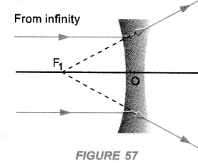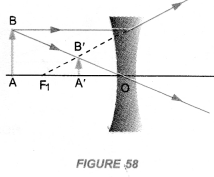Question 34.
A ray of light, incident obliquely on a face of a rectangular glass slab placed in air, emerges from the opposite face parallel to the incident ray. Draw labelled diagram to show it. State two factors on which the lateral displacement of the emergent ray depends. [CBSE (Foreign) 2008, 2013]
Perform an experiment to demonstrate that light bends from its path, when it falls obliquely on the surface of a glass slab. Also show that angle of incidence is about equal to the emergent angle.

1. Take a glass slab and place it on a white sheet of paper fixed on a drawing board.
2. Mark the boundary ABCD of the glass slab.
3. Fix two pins P1 and P2, vertically on the drawing board such that line joining the pins is inclined to the edge AB of the glass slab (Figure 37).4. Now, look through the glass slab from opposite side so that the images of pins P1 and P2 are seen exactly in line. Fix two pins P3 and P4, vertically on the drawing board such that pins P3, P4 and image of P1 and P2 are exactly in one line.
5. Remove the glass slab and all pins. Join the points P1 and P2 with a line and extend this line to touch the edge AB at P. Similarly join the points P3 and P4 with a line and extend the line to touch the edge DC at Q.
6. Join points P and Q with a straight line.
7. Draw normal NN’ at P on the edge AB and a normal N1N1‘ at Q on the edge DC.
8. Measure ∠P2 PN and ∠P3 QN1‘ using a protractor or dee.

This experiment shows that when light falls obliquely on a glass slab, it bends along PQ from its original path along PL.
Also ∠P2PN ≈ ∠P3QN1‘. That is, angle of incidence is about equal to the emergent angle.
Note : Distance between pins P1 and P2, and between P3 and P4 must be large.

Question 35.
A convex lens is placed in contact with a concave lens so that the power of the combination is positive. Name the type of the lens, this combination of lenses behaves.
Power of a convex lens is positive and the power of a concave lens is negative. Since the power of combination of lenses is positive, so the combination of lenses behaves as a convex lens.

Question 36.
An object is placed at the following distances from a convex lens of focal length 15 cm.
(i) 35 cm
(ii) 30 cm
(iii) 20 cm
(iv) 10 cm
Which position of the object will produce an image of same size as that of the object ? (CBSE 2011)
When object is placed at 2 F i.e at 2 f= 30 cm from a convex lens, then the image of same size as that of the object is formed.

Question 37.
A lens X has focal length 20 cm and lens Y has focal length 40 cm. Which lens would you select to obtain a more convergent beam of light ? (CBSE 2011)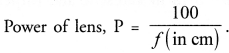Thus, a lens of less focal length has large power of converging a parallel beam (in cm) of light. Therefore, lens X will be used to obtain a more convergent beam of light.

Long Answer Questions ( 5 Marks)

Question 1.
A thin converging lens forms a :
(i) real magnified image
(ii) virtual magnified image of an object placed in front of it.
(a) Write the positions of the objects in each case.
(b) Draw labelled diagrams to show the image formation in each case.
(c) How will the following be affected on cutting this lens into two halves along the principal axis ?

1. focal length,
2. intensity of the image formed by half lens. (CBSE 2011)

(a)

1. A converging or convex lens forms real and magnified image of an object, when the object is placed between F1 and 2F1
2. A converging lens forms a virtual magnified image of an object, when the object is placed between the focus and optical centre of the converging lens.

(b)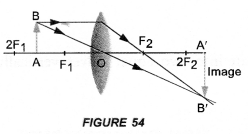(c)

1. Focal length of each half will be equal to the focal length of the lens. If converging lens of focal length f is cut into two equal halves as shown in figure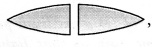then the focal length of each half = f.
2. Intensity of the image formed ∝ (aperture of the lens)2. Aperture of each cut half of the lens is ½ times aperture of the lens. Hence, intensity of the image formed by half lens will decrease.

Question 2.
For the given data showing object distance and focal length of three concave mirrors, answer the following questions :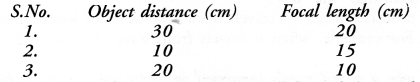1. Out of the three in which case the mirror will form the image having same size as the object ?
2. Which mirror is being used as a make-up-mirror ?
3. Draw the ray diagram for part (1) and part (2) (CBSE 2011, 2015)

3.